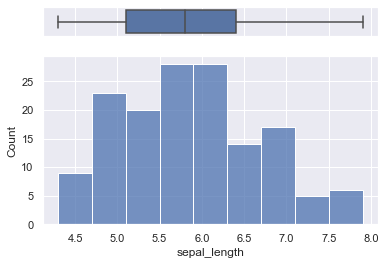Adding a boxplot on top of a histogram can help you in better understanding the distribution of the data and visualizing outliers as well as quartiles positions. Note that we chose to use the histplot function below, though you could definitely use the distplot function also.

``````# libraries & dataset
import seaborn as sns
import matplotlib.pyplot as plt
# set a grey background (use sns.set_theme() if seaborn version 0.11.0 or above)
sns.set(style="darkgrid")

# creating a figure composed of two matplotlib.Axes objects (ax_box and ax_hist)
f, (ax_box, ax_hist) = plt.subplots(2, sharex=True, gridspec_kw={"height_ratios": (.15, .85)})

# assigning a graph to each ax
sns.boxplot(df["sepal_length"], orient="h", ax=ax_box)
sns.histplot(data=df, x="sepal_length", ax=ax_hist)

# Remove x axis name for the boxplot
ax_box.set(xlabel='')
plt.show()``````## Contact & Edit

👋 This document is a work by Yan Holtz. You can contribute on github, send me a feedback on twitter or subscribe to the newsletter to know when new examples are published! 🔥